Sie sind hier: ICP » R. Hilfer » Publikationen

# 8 Fractional Time Evolution

[page 214, §1]
[214.1.1] The induced time evolution is obtained from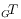by iteration. [214.1.2] According to its definition in eq. (16) the induced measure preserving transformationacts as a convolution in time,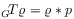(17)

where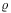is a mixed state on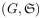. [214.1.3] Iterating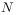times gives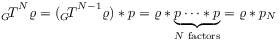(18)

where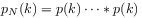is the probability density of the sum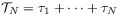(19)

ofindependent and identically with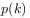distributed random recurrence times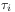. [214.1.4] Then the long time limit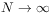for induced measure preserving transformations on subsets of small measure is generally governed by well known local limit theorems for convolutions [42, 43, 44, 45]. [214.1.5] Application to the case at hand yields the following fundamental theorem of fractional dynamics [1, 21]

###### Theorem 8.1.

Assume that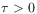is maximal in the sense that there is no larger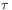for which all recurrence times lie in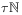. [214.1.6] Then the following conditions are equivalent:

1. [214.1.7] Either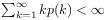or there exists a number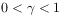such that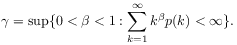(20)
2. [214.1.8] There exist constants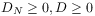and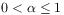such that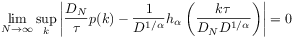(21)

where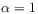, if, and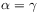otherwise. [214.1.9] The function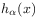vanishes for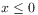, and is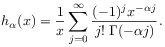(22)

for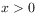.

[page 215, §0]    [215.0.1] If the limit exists, and is nondegenerate, i.e.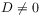, then the rescaling constants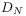have the form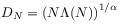(23)

where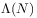is a slowly varying function , i.e.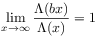(24)

for all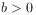.

[215.1.1] The theorem shows that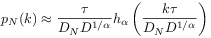(25)

holds for sufficiently large. [215.1.2] The asymptotic behaviour of the iterated induced measure preserving transformation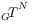forallows to remove the discretization, and to find the induced continuous time evolution on subsets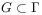. [215.1.3] First, the definition eq. (15) is extended from the arithmetic progression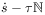to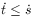by linear interpolation. [215.1.4] Let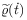denote the extended measure defined for. [215.1.5] Using eq. (11) and setting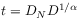(26)

the summation in eq. (18) can be approximated for sufficently largeby an integral. [215.1.6] Then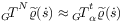, where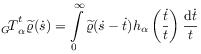(27)

is the induced continuous time evolution.[215.1.7]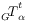is also called fractional time evolution. [215.1.8] Laplace tranformation shows thatfulfills eq. (5). [215.1.9] It is an example of subordination of semigroups [47, 33, 7, 48]. [215.1.10] Indeed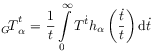(28)

where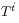denotes right translations on the interpolated measure. [215.1.11] Because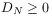and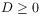, eq. (26) implies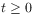. [215.1.12] As remarked in the introduction, the induced time evolution is in general not a translation (group or semigroup), but a convolution semigroup. [215.1.13] The fundamental classification parameter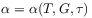(29)

[page 216, §0]    depends not only on the dynamical rule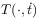and the subset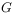, but also on the discretization time step, i.e. on the time scale of interest.